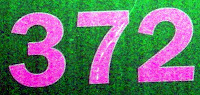## Thursday, April 8, 2010

### 372

372 = 22 x 3 x 31.

372 has a unique representation as a sum of three squares: 372 = 42 + 102 + 162.

372 is a hexagonal pyramidal number, having the form n(n + 1)(4n - 1)/6 for n = 8.

372/(3 + 7 + 2) is a prime number, 31.

101,556 = 273 x 372 = 651 x 156.NGC 372 is a triple star in the constellation Pisces.